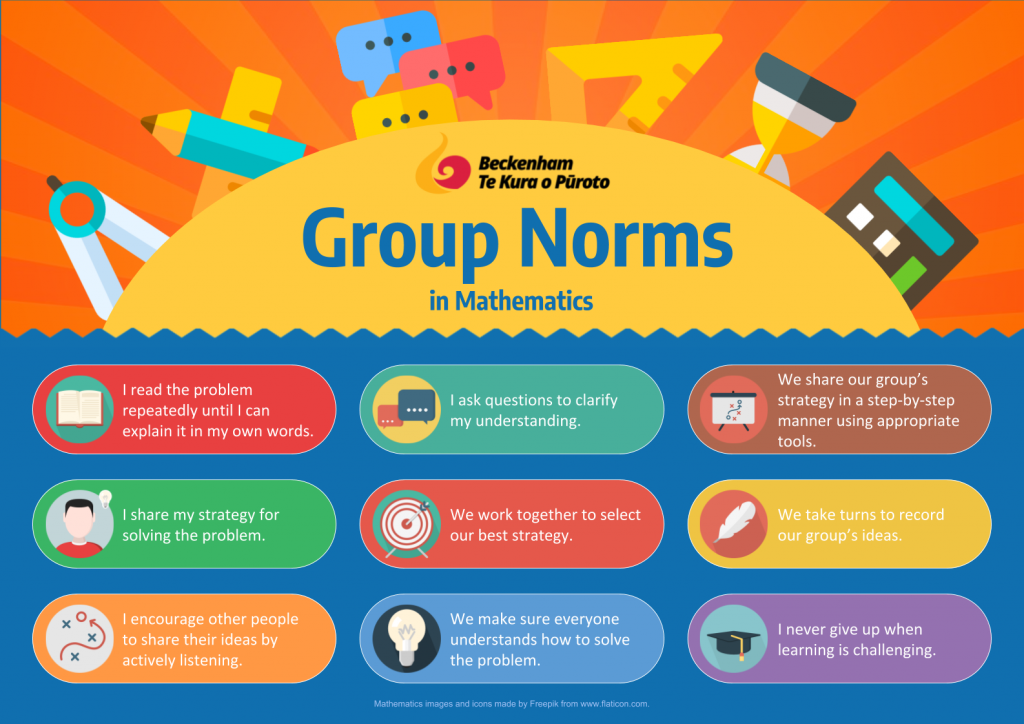# Mathematics

## How do Maths Lessons Operate at our School?

Each student is in a maths group. Throughout the week each group rotates through a variety of activities such as:

• Group problem-solving lessons.
• Teacher-led lessons.
• Follow-up activities.
• Basic facts practice.

## Group Problem-Solving Lessons

Group problem-solving is a new part of our mathematics programme. Its purpose is to raise student achievement, increase agency, increase social skills, enhance mathematical dispositions and enhance the valuing of mathematics within the home and cultural contexts. It is broken up into four stages.

### 1. The Launch

Group lessons start with a challenging, real-world problem that the teacher shares with the students. During the launch stage, the students try to understand what the problem is about (the context) and what it is asking them to find out.

### 2. Group Work

The students work in small groups to try to solve the problem. During this time, they practise the following norms:### 3. Sharing

Near the end of the lesson, the groups come back together. Teachers select and sequence groups to share their thinking. The students then share their strategies for solving the problem. Different groups may have used different strategies.

Students are encouraged to question and challenge the strategies presented to them. They are encouraged to justify their thinking by explaining ‘why’.

### 4. Connect

At the end of the lesson, the teacher makes sure that the students are able to make connections between the problem and the important mathematical idea that the lesson was based on. These connections may be (but are not limited to):

• Identifying the similarities and differences between the strategies used by different groups.
• Generalising (looking for rules and relationships).
• Correcting misconceptions and errors.
• Teaching new strategies or knowledge.

## Basic Facts

We use teach basic facts (e.g. multiplication tables, addition facts etc.) in a variety of ways. Here are some helpful websites that students can use to improve their basic facts knowledge at home:

• Mathematics Games – helps students learning addition, subtraction, multiplication and division facts.